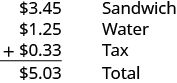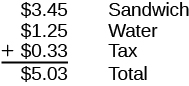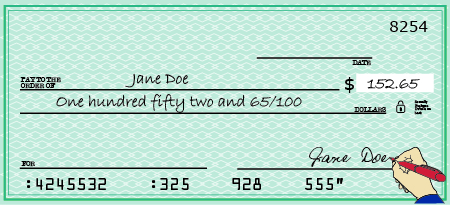## Writing and Naming Decimals

### Learning Outcomes

• Name decimals
• Write decimals

You probably already know quite a bit about decimals based on your experience with money. Suppose you buy a sandwich and a bottle of water for lunch. If the sandwich costs $\text{\3.45}$ , the bottle of water costs $\text{\1.25}$ , and the total sales tax is $\text{\0.33}$ , what is the total cost of your lunch?The total is $5.03$. Suppose you pay with a $5$ bill and $3$ pennies. Should you wait for change? No, $\text{\5}$ and $3$ pennies is the same as $\text{\5.03}$.

Because $\text{100 pennies}=\text{\1}$, each penny is worth ${\Large\frac{1}{100}}$ of a dollar. We write the value of one penny as $0.01$, since $0.01={\Large\frac{1}{100}}$.

Writing a number with a decimal is known as decimal notation. It is a way of showing parts of a whole when the whole is a power of ten. In other words, decimals are another way of writing fractions whose denominators are powers of ten. Just as the counting numbers are based on powers of ten, decimals are based on powers of ten. The table below shows the counting numbers.

Counting number Name
$1$ One
$10=10$ Ten
$10\cdot 10=100$ One hundred
$10\cdot 10\cdot 10=1000$ One thousand
$10\cdot 10\cdot 10\cdot 10=10,000$ Ten thousand

How are decimals related to fractions? The table below shows the relation.

Decimal Fraction Name
$0.1$ ${\Large\frac{1}{10}}$ One tenth
$0.01$ ${\Large\frac{1}{100}}$ One hundredth
$0.001$ ${\Large\frac{1}{1,000}}$ One thousandth
$0.0001$ ${\Large\frac{1}{10,000}}$ One ten-thousandth

When we name a whole number, the name corresponds to the place value based on the powers of ten. In Whole Numbers, we learned to read $10,000$ as ten thousand. Likewise, the names of the decimal places correspond to their fraction values. Notice how the place value names in the first table relate to the names of the fractions from the second table.

This chart illustrates place values to the left and right of the decimal point.Notice two important facts shown in the tables.

• The “th” at the end of the name means the number is a fraction. “One thousand” is a number larger than one, but “one thousandth” is a number smaller than one.
• The tenths place is the first place to the right of the decimal, but the tens place is two places to the left of the decimal.

Remember that $5.03$ lunch? We read $5.03$ as five dollars and three cents. Naming decimals (those that don’t represent money) is done in a similar way. We read the number $5.03$ as five and three hundredths.
We sometimes need to translate a number written in decimal notation into words. As shown in the image below, we write the amount on a check in both words and numbers.

When we write a check, we write the amount as a decimal number as well as in words. The bank looks at the check to make sure both numbers match. This helps prevent errors.Let’s try naming a decimal, such as $15.68$. We start by naming the number to the left of the decimal. fifteen______ We use the word “and” to indicate the decimal point. fifteen and_____ Then we name the number to the right of the decimal point as if it were a whole number. fifteen and sixty-eight_____ Last, name the decimal place of the last digit. fifteen and sixty-eight hundredths

The number $15.68$ is read fifteen and sixty-eight hundredths.

### Name a decimal number.

• Name the number to the left of the decimal point.
• Write “and” for the decimal point.
• Name the “number” part to the right of the decimal point as if it were a whole number.
• Name the decimal place of the last digit.

### Exercises

Name each decimal:

1. $4.3$

2. $2.45$

3. $0.009$

4. $-15.571$

Solution

 1. $4.3$ Name the number to the left of the decimal point. four_____ Write “and” for the decimal point. four and_____ Name the number to the right of the decimal point as if it were a whole number. four and three_____ Name the decimal place of the last digit. four and three tenths
 2. $2.45$ Name the number to the left of the decimal point. two_____ Write “and” for the decimal point. two and_____ Name the number to the right of the decimal point as if it were a whole number. two and forty-five_____ Name the decimal place of the last digit. two and forty-five hundredths
 3. $0.009$ Name the number to the left of the decimal point. Zero is the number to the left of the decimal; it is not included in the name. Name the number to the right of the decimal point as if it were a whole number. nine_____ Name the decimal place of the last digit. nine thousandths
 4. $-15.571$ Name the number to the left of the decimal point. negative fifteen Write “and” for the decimal point. negative fifteen and_____ Name the number to the right of the decimal point as if it were a whole number. negative fifteen and five hundred seventy-one_____ Name the decimal place of the last digit. negative fifteen and five hundred seventy-one thousandths

### TRY It

Now we will translate the name of a decimal number into decimal notation. We will reverse the procedure we just used.

### example

1. Write the number six and seventeen hundredths:

 six and seventeen hundredths The word and tells us to place a decimal point. ___.___ The word before and is the whole number; write it to the left of the decimal point. $6$._____ The decimal part is seventeen hundredths. Mark two places to the right of the decimal point for hundredths. $6$._ _ Write the numerals for seventeen in the places marked. $6.17$

2. Write fourteen and thirty-seven hundredths as a decimal.

### try it

In the following video we show more examples of how to write the name of a decimal using a place value chart.

### Write a decimal number from its name.

1. Look for the word “and”—it locates the decimal point.
2. Mark the number of decimal places needed to the right of the decimal point by noting the place value indicated by the last word.
• Place a decimal point under the word “and.” Translate the words before “and” into the whole number and place it to the left of the decimal point.
• If there is no “and,” write a “0” with a decimal point to its right.
3. Translate the words after “and” into the number to the right of the decimal point. Write the number in the spaces—putting the final digit in the last place.
4. Fill in zeros for place holders as needed.

The second bullet in Step 1 is needed for decimals that have no whole number part, like ‘nine thousandths’. We recognize them by the words that indicate the place value after the decimal – such as ‘tenths’ or ‘hundredths.’ Since there is no whole number, there is no ‘and.’ We start by placing a zero to the left of the decimal and continue by filling in the numbers to the right, as we did above.

### example

Write twenty-four thousandths as a decimal.

### try it

In the next video we will show more examples of how to write a decimal given its name in words.

Before we move on to our next objective, think about money again. We know that $1$ is the same as $1.00$. The way we write $1\left(\text{or }1.00\right)$ depends on the context. In the same way, integers can be written as decimals with as many zeros as needed to the right of the decimal.

$\begin{array}{cccc}5=5.0\hfill & & & -2=-2.0\hfill \\ 5=5.00\hfill & & & -2=-2.00\hfill \\ 5=5.000\hfill & & & -2=-2.000\hfill \end{array}$
and so on $\dots$

## Contribute!

Did you have an idea for improving this content? We’d love your input.